• 矩阵和标量、向量相加
2021-11-21 00:37:11

# 矩阵和标量、向量相加

在线性代数中，只有相同维数的矩阵可以相加，相同维数的向量可以相加，而没有定义矩阵与标量、向量的加法。

在深度学习中，我们允许矩阵与标量、向量相加，产生另一个矩阵。

## 矩阵与标量相加

C = A + b , C i , j = A i , j + b \bold{C} = \bold{A} + b, \quad C_{i,j} = A_{i,j} + b

即，将标量加到矩阵的每个元素上。

numpy中有如下输入输出：

# [in]
a = np.ones((3, 3))
b = 1
a + b

# [out]
array([[2., 2., 2.],
[2., 2., 2.],
[2., 2., 2.]])


## 矩阵与向量相加

C = A + b \bold{C} = \bold{A} + \bold{b}

一般情况下，我们所称的向量为列向量，即
b = [ b 1 ⋮ b n ] \bold{b} = \begin{bmatrix} b_1 \\ \vdots \\ b_n \end{bmatrix}
为了便于排版，一般将其写成 b = [ b 1 , … , b n ] T \bold{b} = [b_1, \dots, b_n]^{T} 的形式。一般我们称 d = [ d 1 , … , d n ] \bold{d} = [d_1, \dots, d_n] 形式的向量为行向量。矩阵与向量相加时，需要区分是与列向量相加还是与行向量相加。

矩阵与列向量相加
C = A + b , C i , j = A i , j + b i \bold{C} = \bold{A} + \bold{b}, \quad C_{i,j} = A_{i,j} + b_i
即，将列向量加到矩阵每一列上。

numpy中有如下输入输出：

# [in]
a = np.ones((3, 3))
b = np.array([1, 2, 3]).reshape(-1, 1)
a + b

# [out]
array([[2., 2., 2.],
[3., 3., 3.],
[4., 4., 4.]])


矩阵与行向量相加
C = A + b , C i , j = A i , j + b j \bold{C} = \bold{A} + \bold{b}, \quad C_{i,j} = A_{i,j} + b_j
即，将行向量加到矩阵每一行上。

numpy中有如下输入输出：

# [in]
a = np.ones((3, 3))
b = np.array([1, 2, 3]).reshape(1, -1)
a + b

# [out]
array([[2., 3., 4.],
[2., 3., 4.],
[2., 3., 4.]])机器学习
更多相关内容
• 关于Numpy中的行向量列向量详解行向量方式1import numpy as npb=np.array([1,2,3]).reshape((1,-1))print(b,b.shape)结果：(array([[1, 2, 3]]), (1, 3))方式2import numpy as npb=np.array([[1,2,3]]) #两层'[]'...

关于Numpy中的行向量和列向量详解

行向量

方式1

import numpy as np

b=np.array([1,2,3]).reshape((1,-1))

print(b,b.shape)

结果：

(array([[1, 2, 3]]), (1, 3))

方式2

import numpy as np

b=np.array([[1,2,3]]) #两层'[]'

print(b,b.shape)

结果

(array([[1, 2, 3]]), (1, 3))

列向量

方式1

import numpy as np

a=np.array([1,2,3]).reshape((-1,1))

print(a,a.shape)

结果：

(array([,

,

]), (3, 1))

方式2

import numpy as np

a=np.array([[1,2,3]]).T

print(a,a.shape)

结果

(array([,

,

]), (3, 1))

以上这篇关于Numpy中的行向量和列向量详解就是小编分享给大家的全部内容了，希望能给大家一个参考，也希望大家多多支持我们。

时间： 2019-11-29

有点抱歉的是我的数学功底确实是不好,经过了高中的紧张到了大学之后松散了下来.原本高中就有点拖后腿的数学到了大学之后更是一落千丈.线性代数直接没有学明白,同样没有学明白的还有概率及统计以及复变函数.时至今日,我依然觉得这是人生中让人羞愧的一件事儿.不过,好在我还有机会,为了不敷衍而去学习一下. 矩阵的转置有什么作用,我真是不知道了,今天总结完矩阵转置的操作之后先去网络上补充一下相关的知识. 今天的代码操作如下: In : arr1 = np.arange(20) In : arr1

np.newaxis 新增一个轴 如何将数组[0,1,2]转换成列向量 用ndarray[: , np.newaxis] 代码实质就是将原本的(0,1,2)移到行上,然后新增一列 其实可以更简单 ndarray.shape=(3,1) >> x = np.arange(3) >> x array([0, 1, 2]) >> x[:, np.newaxis] array([, , ]) >> x[:, None] array([, [1

np.linalg.norm(求范数):linalg=linear(线性)+algebra(代数),norm则表示范数. 函数参数 x_norm=np.linalg.norm(x, ord=None, axis=None, keepdims=False) ①x: 表示矩阵(也可以是一维) ②ord:范数类型 向量的范数: 矩阵的范数: ord=1:列和的最大值 ord=2:|λE-ATA|=0,求特征值,然后求最大特征值得算术平方根 ord=∞:行和的最大值 ③axis:处理类型 axis=1表

在下面的代码里面,我们利用numpy和scipy做了很多工作,每一行都有注释,讲解了对应的向量/矩阵操作. 归纳一下,下面的代码主要做了这些事: 创建一个向量 创建一个矩阵 创建一个稀疏矩阵 选择元素 展示一个矩阵的属性 对多个元素同时应用某种操作 找到最大值和最小值 计算平均值.方差和标准差 矩阵变形 转置向量或矩阵 展开一个矩阵 计算矩阵的秩 计算行列式 获取矩阵的对角线元素 计算矩阵的迹 计算特征值和特征向量 计算点积 矩阵的相加相减 矩阵的乘法 计算矩阵的逆 一起来看代码吧: # 加载n

如下所示: >>> import numpy as np >>> a = np.array([[1, 2, 3], [3, 1, 2]]) >>> b = np.array([[5, 2, 6], [5, 1, 2]]) >>> a array([[1, 2, 3], [3, 1, 2]]) >>> b array([[5, 2, 6], [5, 1, 2]]) >>> c = a + b >

在PCA中有遇到,在这里记录一下 计算矩阵的特征值个特征向量,下面给出几个示例代码: 在使用前需要单独import一下 >>> from numpy import linalg as LA >>> w, v = LA.eig(np.diag((1, 2, 3))) >>> w; v array([ 1., 2., 3.]) array([[ 1., 0., 0.], [ 0., 1., 0.], [ 0., 0., 1.]]) >>>

直接使用Python来实现向量的相加 # -*-coding:utf-8-*- #向量相加 def pythonsum(n): a = range(n) b = range(n) c = [] for i in range(len(a)): a[i] = i**2 b[i] = i**3 c.append(a[i]+b[i]) return a,b,c print pythonsum(4),type(pythonsum(4)) for arg in pythonsum(4): print arg在用pandas包和numpy包对数据进行分析和计算时,经常用到DataFrame和array类型的数据.在对DataFrame类型的数据进行处理时,需要将其转换成array类型,是以下列出了三种转换方法. 首先导入numpy模块.pandas模块.创建一个DataFrame类型数据df import numpy as np import pandas as pd df=pd.DataFrame({'A':[1,2,3],'B':[4,5,6],'C':[7,8,9]}) 1.使用DataFra

浅谈NumPy中的维度Axis NumPy中的维度是一个很重要的概念,很多函数的参数都需要给定维度Axis,如何直观的理解维度呢?我们首先以二维数组为例进行说明,然后推广到多维数组. (有人将ndim属性叫维度,将axis叫轴,我还是习惯将axis称之为维度,axis=0称为第一个维度) 二维数组的列子 下面是一个二维数组的列子: In : import numpy as np In : x = np.random.randint(0, 9, (2, 3)) In : x OuPython矩阵的基本用法 mat()函数将目标数据的类型转化成矩阵(matrix) 1,mat()函数和array()函数的区别 Numpy函数库中存在两种不同的数据类型(矩阵matrix和数组array),都可以用于处理行列表示的数字元素,虽然他们看起来很相似,但是在这两个数据类型上执行相同的数学运算可能得到不同的结果,其中Numpy函数库中的matrix与MATLAB中matrices等价. 直接看一个例子: import numpy as np a = np.mat('1 3;5 7')

如果用到数据筛选功能,可以使用x if condition else y的逻辑实现.如果使用的是纯Python,可以使用不断迭代的方式对每一组元素组合进行相应的判断筛选.不过,如果使用numpy中的向量化计可以大大加速运算的过程. 在numpy中有一个这个三目运算的向量版本numpy.where.where方法可以接收三个参数,第一个参数为条件向量,而第二.第三个参数可以是矩阵也可以是标量.接下来做一下相应功能的纯Python功能实现以及向量方式实现. 记录如下: In : xarr =

本文先比较range与arange的异同点,再详细介绍各自的用法,然后列举了几个简单的示例,最后对xrange进行了简单的说明. 1. range与arange的比较 (1)相同点:A.参数的可选性.默认缺省值是一样的:B.结果均包括开始值,不包括结束值: C.arange的参数为整数是,与range函数等价:D.都具备索引查找.要素截取等操作. (2)不同点:A.range函数的参数只能为整数,arange的参数为数值型,包括整数和浮点数: B.输出的数据类型不同,range的输出为列表(li

nonzero函数返回非零元素的目录. 返回值为元组, 两个值分别为两个维度, 包含了相应维度上非零元素的目录值. import numpy as np A = np.mat([[0,1,2,3,4,3,2,1,0],[0,1,2,3,4,5,6,7,0]]) x = A.nonzero() #取出矩阵中的非零元素的坐标 print x #输出是一个元组,两个维度.一一对应, #返回非零元素在矩阵中的位置,前一个列表存放非零行坐标,后一个列表存放非零元素列坐标 #(array([0, 0, 0,

1.列表 list是处理一组有序项目的数据结构,即你可以在一个列表中存储一个序列的项目.列表中的项目.列表中的项目应该包括在方括号中,这样python就知道你是在指明一个列表.一旦你创建了一个列表,你就可以添加,删除,或者是搜索列表中的项目.由于你可以增加或删除项目,我们说列表是可变的数据类型,即这种类型是可以被改变的,并且列表是可以嵌套的. 列表是可以改变的,能够增加或减少,(append和del函数) 2.元组 元祖和列表十分相似,不过元组是不可变的.即你不能修改元组.元组通过圆括号中用逗号

展开全文• 最近,我在用MATLAB画一个三维图的时候,发现了MATLAB中行向量与列向量相加的神奇机制. 在使用MATLAB画三维图时,函数要求因变量z必须为一个行与列都x,y严格相关的矩阵.一般都是用meshgrid函数生成两个维数相同的矩阵...

最近,我在用MATLAB画一个三维图的时候,发现了MATLAB中行向量与列向量相加的神奇机制.

在使用MATLAB画三维图时,函数要求因变量z必须为一个行与列都与x,y严格相关的矩阵.一般都是用meshgrid函数生成两个维数相同的矩阵后,在此基础上算出z.但由于我初学MATLAB 一开始不知道meshgrid函数和z的要求,一阵骚操作发现了和meshgrid等效的一个方法.

定义了一个1x2向量X和一个1x3向量Y.meshgrid函数把X这个行向量复制出了length(Y)份后组装成一个矩阵赋给了X_new,又把Y转置为列向量后复制出了length(X)份后组装成一个矩阵赋给了Y_new.最终得到的两个新的矩阵均为3x2矩阵,行数与length(Y)相等,列数与length(X)相等.

再让X与Y相加后得到Z.这样就能利用mesh函数画出三维图了.而当我直接令Z = X+Y’时,得到的Z居然和通过meshgrid方法最终得到的Z一样.并且可生成与meshgrid方法一样的三维图询问老师后得知,旧版本(2018之前)的MATLAB是没有这个机制的.在新版本中,只要让行列向量相加,行向量扩充为length(列向量)个,每一行的每个元素都会加上列向量中对应行的那个数.

如下图,Z = X’+Y注意的是若想利用此方法直接得到能画出三维图的Z,需要转置第二个行向量(Y).转置第一个行向量(X)得到的结果与meshgrid不一样,也不符合mesh函数的要求.

本文仅记录笔者的一个发现,还是建议大家用meshgrid函数.

展开全文matlab
• 在之前的文章中，我们已经解释了向量可以写成[1 x 3]的矩阵（一）。但是现在也可以写成[3 x 1]（三）的形式。从技术上来说，这两种表示点和向量的方式都是合理的，选用一种模式只是涉及到习惯的问题。 ...

在之前的文章中，我们已经解释了向量可以写成[1 x 3]的矩阵（一行三列）。但是现在也可以写成[3 x 1]（三行一列）的形式。从技术上来说，这两种表示点和向量的方式都是合理的，选用一种模式只是涉及到习惯的问题。

向量写成[1 x 3]的矩阵 V = [x  y  z]

向量写成[3 x 1]的矩阵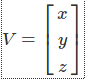在第一个例子中，我们写了一个[1 x 3]的矩阵，我们称之为行顺序（row-major order）：向量被写作一行三个数据。在第二个例子中，我们称点或者是向量为列顺序(column-major order)：点或者是向量的三个坐标被写成一列。

记住我们表达点或者是向量使用矩阵去乘以一个[3 x 3]的变换矩阵（为了简单起见我们使用[3 x 3]的矩阵而不是[4 x 4]的矩阵）。我们也学习到只有左边矩阵的列数等于右边矩阵的行数的时候这两个矩阵才可以相乘。换句话说也就是[m x p][ p x n]的两个矩阵可以相乘，而[p x m][p x n]的两个矩阵不可以相乘。记住我们可以将一个向量写成[1 x 3]，那么它可以和一个[3 x 3]的矩阵相乘，但是如果我们把这个向量写成[3 x 1]那么我们不能和一个[3 x 3]的矩阵相乘。这个道理会在下面的例子中显示。绿色的维数是一样的所以这个乘积是合法的（所得到的结果就是一个变换的点的坐标，它的形式是一个[1 x 3]的矩阵）：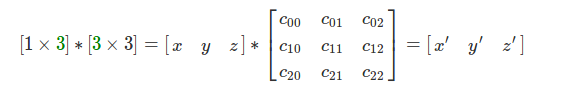里面的维数为(1和3)的两个矩阵在乘法中国因此这个成绩是不可能实现的：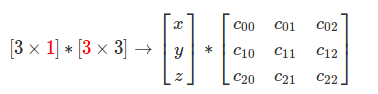那么我们应该怎么办？这个问题的答案不在是向量或者点去乘以矩阵，而是矩阵M去乘以向量V。换句话说，我们将点移动，或者向量移动到乘法的右边：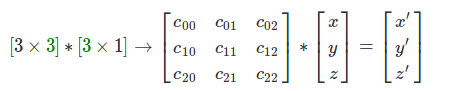记住这个操作所得到的移动的点写成[3 x 1]的矩阵方式。因此我们可以用我们想要的方式去获得一个变换的点。问题解决了，总结一下，当习惯的时候我们表达点或者向量是用的行顺序[1 x 3]，我们需要将点防止在乘法的左边，[3 x 3]的矩阵应该放置在乘法的右边。在数学上，这叫做左或者提前乘法（pre-multiplication）.如果你决定将向量写成列顺序，那么点应该放置在乘法的右边，这个叫做右或者是后置乘法（post-multiplication）.

我们应该对这些东西的使用格外的小心。比如，在Maya中，矩阵是后置乘法的，比如将一个点p从物体坐标系中移动到世界坐标系中，你需要的是后置的乘法P = P x WM.这是很迷惑人的，因为他实际上是提前乘法，但是他们说这个是以矩阵的位置来确定这个乘法的。在术语上这个实际上是不准确的。在Maya中，点和向量应该表达称行顺序，因此它们是属于提前乘法的（意味着点和向量出现在矩阵的之前）。

下面的这个表格总结了这两种惯例的不同之处（P表示点，V表示向量，M代表矩阵）。

行顺序  P / V = [x y z] 左或者前置乘法  P / V * M

列顺序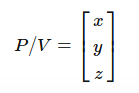右或者是后置乘法  M x P / V

现在我们学习了两种常规表达方式，你可能会问，为什么不把它们写在纸上？我们知道如何计算两个向量的乘积：A向量的当前行乘以相对应的B向量的当前列然后将这些结果相加。让我们运用这个公司去比较它们的结果：

行顺序（row-major order）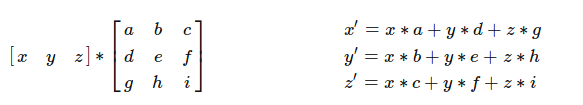列顺序（column-major order）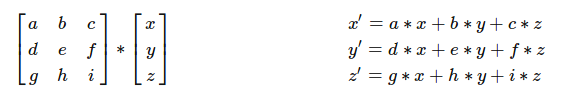点或者是向量和矩阵相乘不论是用行顺序还是列顺序我们都应该得到相同的结果。如果你在一个3D应用中，我们将一个点绕Z轴旋转一定的角度，你期望通过旋转之后的点在确定的位置上，不论开发者是用什么样的的方式表达点或者向量。但是，当你看到上面的表格之后，乘以一个行顺序或者是列顺序的矩阵我们不会得到相同的结果。回到我们的原点中，我们需要转置一个[3 x 3]的矩阵使它用在列向量中，从而确保x', y', z'是一样的结（如果你需要知道矩阵的转置是什么，请看矩阵操作章节），这里我们得到的：

行顺序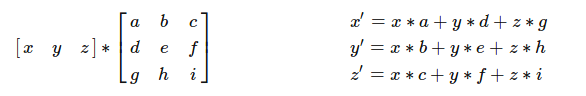列顺序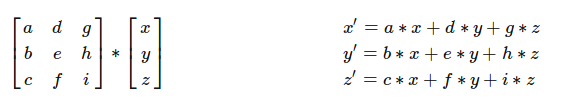总结来说，从行顺序到列顺序中不仅仅是变换了点和向量相对于乘法的位置而且将[3 x 3]的矩阵转置，这样做确保两个得到的结果是一样的。

从这些观察中可以得到，你可以将一系列的变换应用于一个点，当你使用行顺序的时候它们可以被写成一个阅读的顺序。比如你想将点P通过点T平移，然后绕Z轴旋转，然后绕y轴旋转。你可以写成下面的样式：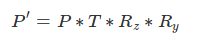如果你使用一个列顺序的话，你需要调用这些变换使用相反的顺序，如下面的样子：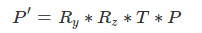因此你可能回想，这里肯定有一个原因更会喜欢一种。实际上，这两种习惯都是正确的，会给我们相同的答案，但是因为一些技术上的原因，数学和物理偏向于使用列向量。

使用列向量进行变换个何如我们写的数学公式。

行矩阵可以使得我们的矩阵更简单，因此对于这个教学网站来说我们使用它（和Maya， DirectX是一样的它们也在标准手册中定义了相关习惯）。但是对于另外一些3D的api,比如opengl， 使用的是列顺序习惯。

（1）隐式编程：它影响性能吗？

这里有一个潜在的很重要的需要考虑的当你使用行顺序或者是列顺序的时候，但是这个对于真正的习惯并没有什么用处，哪个更实用。它有跟多的使用电脑的工作方式。记住我们将会处理[4 x 4]的矩阵，典型的C++实现的矩阵是下面这个样子：

class Matrix44 {
float m
}
你可以看到16个矩阵的参数存储在二维数组里面（如果你想选用双精度的，你可以使用模板类）。那么16个参数会以下面的方式进行排列：c00, c01, c02, c03, c10, c11, c12, c13, c20, c21, c22, c23, c30, c31, c32, c33.换句话说，他们在内存里是连续的。让我们来看看这些向量和矩阵的乘法是怎么样在行顺序中获取的：

// row-major order
x' = x * c00 + y * c10 + z * c20
y' = x * c01 + y * c11 + z * c21
z' = x * c02 + y * c12 + z * c22 
你可以看到这些x'的元素不是线性获取的。换句话说，为了计算x'的值，我们需要第1， 5， 9个矩阵的数值。为了计算y'我们需要获取第2， 6， 10的数值。计算z'我们需要3, 7, 11的数值。在计算的世界里，不是线性第获取数组的元素不是一件好的事情。它实际上利用了cpu的缓存技术。我们不会去深入地研究，但是我们想说的是离cpu最近的缓存获取最快，但是它只能存储有限的数据。当cpu需要获取数据的时候，它首先检出数据是否存在缓存中。如果它存在，cpu就会马上获取他们，如果它不存在，首先cpu需要穿件一个缓存的入口，然后把内存中的数据复制到这里来。这个过程很显然地要比从缓存中直接读取数据花费更多的时间。cpu不仅会复制需要的数据，而且会复制一连串的数据，比如24个字节的数据。因为硬件工程师理解如果你的代码需要获取数组的元素，你更倾向于连续地获取。确实，在程序中，我们经常循环遍历玩数组的元素，这个假设因此是正确的。应用到我们的矩阵问题上，不连续地获取数组中元素当然是个问题。如果cpu允许三个浮点数的缓存，那么我们当前的实现就可能导致缓存没法命中。因为我们用于计算x', y', z'的参数是有5个分离。另一方面说，如果你使用的是列顺序，计算x'的值值需要获取1, 2,3个数值。

// column-major order
x' = c00 * x + c01 * y + c02 * z
y' = c10 * x + c11 * y + c12 * z
z' = c20 * x + c21 * y + c22 * z 
矩阵的参数可以按顺序获取说明我们可以使用cpu的缓存技术。因此从编码的角度来说的话，进行点或者向量矩阵相乘的时候使用列顺序会比使用行向量更好。实际的，我们并没有展示这个例子（当你使用你优先级标志-O -O2 -O3编译你的程序的时候，编译器可以最优化你的循环），我们成功使用了行顺序的版本没有丢失任何的性能，相比较列向量来说。

template<typename T>
class Vec3 {
public:
Vec3(T xx, T yy, T zz) : x(xx), y(yy), z(zz) { }
T x, y, z, w;
};

#include <iostream>

#define ROWMAJOR 1

template<typename T>
class Matrix44 {
public:
T m;
Vec3<T> multVecMatrix(const Vec3<T> &v) {
#ifdef ROWMAJOR
return Vec3<T>(v.x * m + v.y * m + v.z * m,
v.x * m + v.y * m + v.z * m,
v.x * m + v.y * m + v.z * m);
#else
return Vec3<T>(v.x * m + v.y * m + v.z * m,
v.x * m + v.y * m + v.z * m,
v.x * m + v.y * m + v.z * m);
#endif
}
};

#include <cmath>
#include <cstdlib>
#include <cstdio>
#include <ctime>

#define MAX_ITER 10e8

int main(int argc, char **argv) {
clock_t start = clock();
Vec3<float> v(1, 2, 3);
Matrix44<float> M;
float *tmp = &M.m;
for (int i = 0; i < 16; i++)
*(tmp + i) = drand48();
for (int i = 0; i < MAX_ITER; ++i) {
Vec3<float> vt = M.multVecMatrix(v);
}
fprintf(stderr, "Clock time %f\n", (clock() - start) / float(CLOCKS_PER_SEC));
return 0;
}
如果运行的话就会走row分支，因为ROWMAJOR之前已经有定义。

两者运行时间基本上一致

wang@wang:~/workspace/graphic$./multiMatrix Clock time 14.660049 wang@wang:~/workspace/graphic$ g++ -std=c++11 multiMatrix.cpp -o multiMatrix
wang@wang:~/workspace/graphic\$ ./multiMatrix
Clock time 14.597244
列顺序稍微快那么一点点，不到0.1秒的差距。

（2）计算中的行顺序和列顺序

为了完整性，行顺序和列顺序可以用于计算中，用来描述多维数组的元素在内存中的分布。在行顺序中，多维数组的元素排列从左到右，从上到下。可以使用C/c++表示他们。比如，矩阵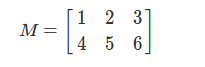可以写成代码的方式：

float m={{1, 2, 3}, {4, 5, 6}}; 
然后这些元素会在内存中线性排列：

1 2 3 4 5 6

在列向量中，它用在Fortran和matlab中，元素的在内存中的排列方式是从上到下，从左到右的。使用上面的矩阵，那么矩阵按照下面的方式存储：

1 4 2 5 3 6

知道矩阵中的元素在内存中是如何存放的是很重要的，因为有时候你会使用指针的偏移去获取他们。对于循环的优化（之前的小节中我们已经讲到它会影响到cpu的缓存性能）。但是，因为我们只考虑c/c++作为我们的编程语言，列顺序我们没有多大的兴趣。我们仅仅只是在计算中提到它们是什么，因此你可能会根据具体的语义去描述它们。你应该不能将它们混合。在数学上，它们描述你是用行向量，还是列向量。在计算上，它们描述了数据的储存或者获取方式。

Opengl是一个很有趣的例子，当GL初始化的时候，开发者选用行向量。开发者开发新功能的时候又要回到列向量中。但是，为了兼容性，它们不想改变点和矩阵的成绩，而是去改变矩阵在内存中存储的顺序。换句话说，opengl存储参数使用的列顺序， 参数m03, m13， m23,使用列向量会有13， 14， 14的下标识，而在行向量中有m30, m31, m32的标识。

（3）总结

下面对行向量和列向量这两种用法进行总结：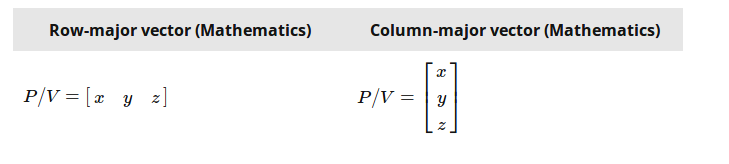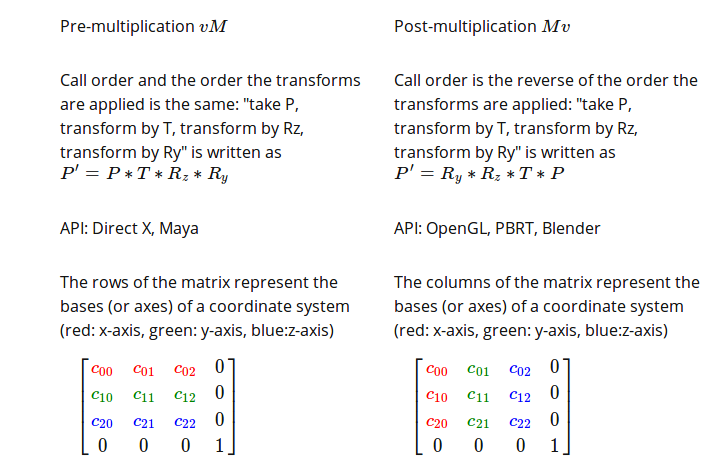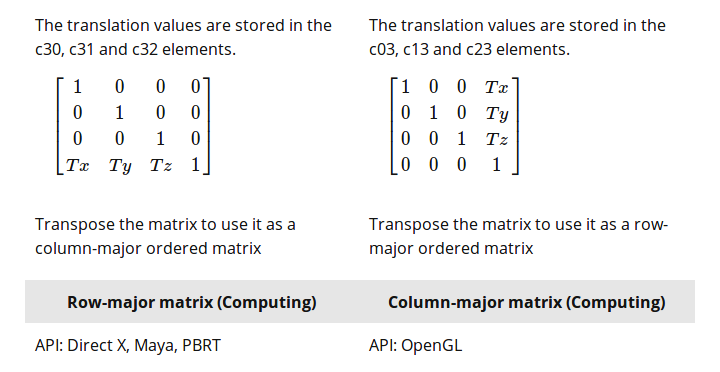上面的英语不难，应该能看懂。

一个肚子在Stackoverflow上提了一个上面表格的问题，认为它很容易使人迷惑。这个话题是迷惑的，尽管我们已经尽了最大的努力，许多人对于它们还是感到迷惑。我们希望我们在Stackoverflow上的回答可以让读者从另一个视角来看待这个问题。

你已经有理论，你现在要做的是用C++实现它，这里有两个不同的问题。

数学上的：你可以些两种方式，可以是列顺序也可以是行顺序，如果使用行顺序，你应该将向量和矩阵的乘法写成vM，这里的表示(1 x 4)的向量，M表示（4 x 4）的矩阵。因为只有写成[1 x 4]*[4 x 4]才能进行矩阵的计算。相似地如果你想使用列顺序，你的向量应该写成竖直的，[4 x 1]，因此矩阵的乘法应该写成这种样式：[4 x 4] *[4 x 1].矩阵防止在向量的前面Mv.前面一种方式叫做后置乘法，后面一种Mv叫做前置乘法（矩阵在前面）。

现在，你需要编一个一个向量或者是点，你需要注意矩阵乘法的顺序，当你把它们写在纸上的时候。如果你使用矩阵T进行平移，然后使用矩阵R进行旋转，然后使用S进行缩放变换，如果使用列矩阵的话，应该写成下面这个样式: v' = S * R * T * v。在行矩阵中你应该写成v' = v * T * R * S.

这是理论上的，我们把它叫做行/列矩阵常用习惯。

计算机上的：这关系到你如果用C++实现它们。好的消息是C++不会给你增加任何的显示。你可以将你的矩阵的参数用你想的方式放置它们，你可以自己些代码表示矩阵的乘法。相似的，你获取向量矩阵乘法的参数的操作也是取决于你。你应该做出一个清晰的区别你的矩阵参数如何放在内存中，以及你将使用那种数学习惯看待你的向量。这两个独立的问题，我们把这个叫做行/列分布。

比如，你可以定义个矩阵类有16个连续的浮点数据。那是好的，这里的参数，m14, m24, m34表示的矩阵平移的(Tx, Ty, Tz).因此这是一种列矩阵，尽然你被告知Opengl使用的矩阵是列矩阵。这里有一个很容易迷惑的就是将参数放置在内存中，不同于你实际想要表达的列矩阵。你写成行的形式，但实际上它们是列的，因此你不知道你时候在做正确的事情。

这里很重要的是，矩阵表示一个坐标系统的三根轴。哪里以及如何存储这些数据完全取决于你。想象一些三个向量表示坐标系统的三根轴，其中它们命名AX(x, y, z), AY(x, y, z), AZ(x, y,z)已经它们的平移向量表达称（Tx, Ty, Tz),那么从数学上你可以使用列向量表示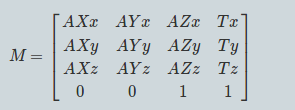这些轴被写成竖直的形式。现在你如果使用行向量，那么可以写成如下的形式：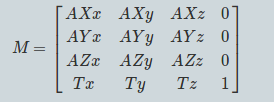它的坐标系统的轴是横向的。因此接下来的问题是你的电脑如何存储这些数据的问题。你可以用下面的形式：

float m = {
AXx, AXy, AXz, 0,
AYx, AYy, AYz, 0,
AZx, AZy, AZz, 0,
Tx, Ty, Tz, 1};
你也可以写成下面这样：

float m = {
AXx, AXy, AXz, Tx,
AYx, AYy, AYz, Ty,
AZx, AZy, AZz, Tz,
0, 0, 0, 1}; 
或者这样：

float m = {
AXx, AYx, AZx, Tx,
AXy, AYy, AZy, Ty,
AXz, AYz, AZz, Tz,
0, 0, 0, 1}; 
再次地高数你，选择什么的数学习惯你随便选择。你仅仅只是将16个参数通过不同的方式存储在内存中，只要你准确地知道它们是什么，因此你可以在后面获取它们。记在心里的是一个向量和一句矩阵相乘应该给你一个相同的行或者是列的数学表达。因此，（x, y, z）和右边矩阵相乘，你需要的知识是怎么存储这些参数在内存中：

Vector3 vecMatMult (
Vector3 v,
float AXx, float AXy, float AXz, float Tx,
float AYx, float AYy, float AYz, float Ty,
float AZx, float AZy, float AZz, float Tz)
{
return Vector3(
v.x * AXx + v.y * AYx + v.z * AZx + Tx,
v.x * AXy + v.y * AYy + v.z * AZy + Ty,
v.x * AXz + v.y * AZz + v.z * AZz + Tz
} 
我们写这个函数的目的是要告诉你不论你用什么的习惯方式，最后你得到的向量和矩阵相乘最终都是向量的输入坐标和坐标系统轴的AX, AY, AZ的相乘之后的叠加（不管你用什么方式，也不管你怎么存储它们），如果你使用：

float m = {
AXx, AXy, AXz, 0,
AYx, AYy, AYz, 0,
AZx, AZy, AZz, 0,
Tx, Ty, Tz, 1}; 
你应该调用

vecMatMult(v, m, m, m, m, m, m, m, m, ... 

如果矩阵为

float m = {
AXx, AYx, AZx, Tx,
AXy, AYy, AZy, Ty,
AXz, AYz, AZz, Tz,
0, 0, 0, 1}; 
你应该调用

vecMatMult(v, m, m, m, m, m, m, m, m, ... 
那告诉你用那种常规形式呢吗？没有，你知识在合适的地方调用了正确的参数当你做向量和矩阵相乘的时候。这是你要知道的所有的，现在到提及到向量和矩阵相乘的时候有些不同，你乘以矩阵的时候，顺序既不是R *S * T, 也不是T * S * R.顺序很重要，现在你用行顺序表达它们：

mt11 = ml11 * mr11 + ml12 * mr21 + ml13 * mr31 + ml14 * mr41
当ml表示左手向量，mr表示右手向量的时候， mt = ml * mr.到那会记住的是我们没有使用[]去获取下标，因为我们不建议去获取存储在1维空间的数组。我们仅仅是讨论写在书本上的矩阵参数。如果你想把这个写成C++，它只取决你怎么像上面那样储存数据。

展开全文• 克隆是指将行向量转换为[1,2,3]入矩阵[[1,2,3][1,2,3][1,2,3]]或列向量，例如进入[[1,1,1][2,2,2][3,3,3]]在matlab或octave中，这很容易做到：x = [1,2,3]a = ones(3,1) * xa =1 2 31 2 31 2 3b = (x') * ones...
• 此函数简化了 Excel 用户向 MATLAB 的过渡。 该函数将通过函数调用添加中的所有元素： x = SUM(A, col);... 这种格式的工作方式 Excel 的“sum()”函数类似，对初学 MATLAB 用户来说非常有用。matlab
• [img=... 如图，看网上教程说python中的numpy有时候有点莫名其妙，于是举了这个例子，我一试，果然是这样。 那请问大佬们，这个该怎么理解呢？以后写代码如何规避这种莫名其妙的bug呢？python
• ## 矩阵与向量的加法

千次阅读 2020-03-17 19:36:15
在Numpy中，矩阵与向量相加时，首先要求即要求矩阵的向量的维数相等。然后就是 矩阵的每一行与向量相加，得出结果。 过程如下图所示： import numpy as np x = np.array([[1, 2, 3], [4, 5, 6],[7,8,9]]) z ...python numpy
• 今天小编就为大家分享一篇python中利用numpy.array()实现俩个数值列表的对应相加方法，具有很好的参考价值，希望对大家有所帮助。一起跟随小编过来看看吧
• numpy 生成矩阵 numpy中可以直接使用matrix生成矩阵X1 = np.matrix([[1,2,3]])X2 = np.matrix([[1,2,3,4]]).T2. 矩阵相加减 (1)如果矩阵是相同... 一个是行向量一个是列向量 b.一个多维矩阵，一个一维矩阵a情况： 行...
• Z=[1 2;3 4] b=sum(Z) %将列相加 c=sum(Z,2) %将行相加 运行结果：  Z =  1 2  3 4 b =  4 6 c =  3  7matlab 矩阵
• ## 向量与行列式笔记

千次阅读 2020-01-12 22:56:26
python实现 1，向量和行列式一，向量1,向量的表示2,维度和分量3，零向量和单位向量 向量是指具有大小和方向的量，在物理学中，通常将向量称为矢量 标量是指只有大小的量，在物理学中，也叫做标量 箭头的方向表示...
• 在R语言中，不同长度的向量也是可以相加和相乘的，乘法的规则和加法类似1，相同长度的向量相加> x> y> z> z 2 4 6 8规则就是 x+y，x+y，x+y，x+y> x> y> z> z[1...
• ## 向量与矩阵乘法

万次阅读 多人点赞 2019-04-02 10:47:20
1. 向量矩阵的本质 1.1 向量的本质 1.2 矩阵的本质 2. 向量、矩阵相乘结果 ...3.1.1 B为列向量 3.1.2 B为矩阵或者行向量 3.2 矩阵的向量表达形式 3.2.1 B为列向量 3.2.1 B为矩阵或者行向量 3.2.3 总结矩阵 算法
• n元向量可以写成行向量列向量的形式，二者相差一次转置运算，记为aT≠aa^T \neq aaT​=a 所有n元实向量的集合记作RnR^nRn 一般地，对所有没有指明的向量，都当作列向量，使用ei∈Rne_i \in R^nei​∈Rn表示第i个...数学
• 在Numpy中，矩阵与向量相加时，矩阵的每一行与向量相加，即要求矩阵的向量的维数相等。 import numpy as np x = np.array([[1, 2, 3], [4, 5, 6]]) # array([[1, 2, 3], # [4, 5, 6]]) y = np.array([1, 2]) ...python numpy
• Axis=1 按计算平均值/标准差等等 Axis=0 按计算平均值/标准差等等 Pandas二维数据结构: DataFrame 定义字典,并将这些数据转换成DataFrame 1）输出有序的数据框 注意：因为字典是无序的数据结构，所以最终的输出...
• 假设，如果有两个具有n和m个元素的行向量r1和r2，通过附加这两个向量来创建nxm个元素的行向量r -r = [r1;r2]然而，要做到这一点，这两个向量应该具有相同数量的元素。类似地，可以附加两个列向量c1和c2，其中分别...
• 行向量与列向量 对于通常的教材、论文来说，只要提到向量，都值列向量。 由于横板印刷原因，使用符号，上角标T来表示转制操作从而变成列向量。 例如 等同于 向量的基本运算 向量加法 （5，2）+（2，5）T = ? 从...
• ps:向量是矩阵的特殊形式，当强调()矩阵时，将其写作为向量。 求解规律如下： 标量/矩阵形式的求导： 将分母中的所有元素对标量依次求导，排列顺序分母排列顺序一致。 矩阵/矩阵形式的求导： 将分子中的元素...机器学习 矩阵
• 4.1 数组运算和矩阵运算从外观形状和数据结构来看,二维数组和数学中的矩阵没有区别.但是,矩阵作为一种变换或映射算符的体现,矩阵运算有着明确而严格的...表4.1.1出了两种运算指令形式的实质内涵的异同.4.1.1 数组...
• 向量没有维数，这意味着没有列向量行向量之分。R是一种区分大小写的解释性语言，R语句的分隔符是分号“;”，或换行符，当语句结束时，可以不使用分号“;”，R语言会自动识别语句结束的位置。R语言只支持单行注释，...
• 向量的基本概念一、向量定义和运算n个实数a , a , a 组成的有序数组定 义 1 2 n称为实数域上的n 维向量。记作： (a ,a , a ) (称行向量)1 2 na  1 T a2 或   ...
• c为列向量 (2)利用冒号表达式生成向量 ： a = 1:2:12,b = 1:5 默认间距为1 (3)线性等分向量的生成： y = linspace(x1,x2) %默认生成100维的行向量 y = linspace(x1,x2,n) %生成n维的行向量 (4)向量的...matlab
• 行向量 列向量 MATLAB 行向量： 创建行向量括在方括号中的元素的集合，用空格或逗号分隔的元素。 r = [7 8 9 10 11] 执行上述语句，返回下述结果： r = 7 8 9 10 MATLAB 列向量： 创建列向量括...matlab
• 患难困苦是磨练人格的最高学府。——苏格拉底(公元前470年～公元前399年)Adversity and pinch are the highest institution of higher learning of harden oneself character. —— Socrates(B.C. 470 - B. C. 399...
• 4] b=a' 得到： b = 2 1 4 行向量转化为列向量也是一样的。 【向量也可以相加减，这里不说了】 两个向量可以组合成一个向量： a=[2,3,4] b=[5,6,7] c=[a,b] 得到： c = 2 3 4 5 6 7 横向量列向量都可以。 等差元素...matlab
• 本文首发于知乎专栏：https://zhuanlan.zhihu.com/p/59688569也同步更新于我的个人博客：https://www.cnblogs.com/nickwu/p/12537014.html因为本学期选修了几门统计有关的课，开始学习统计学中普遍使用的R语言。...vector长度
• 向量加减法，向量的点积（乘），向量的叉积（乘） 向量 是用来表示既有大小又有方向的量，不过向量在空间中没有具体的位置，通常用一个加粗的小写字母来表示一个向量，或者不加粗顶上带有小箭头的小写字母来表示 ...计算机图形学
• python 中的向量怎么表示可以用numpy 库 np.mat([[a],[b],[c]]) 表示一个3*1 列向量 (a,b,c)^Tpython方向向量要怎么表示向量本身属于矢量，有大小也有方向，大小就称为向量的模，向量的方向就是指坐标原点到该向量......